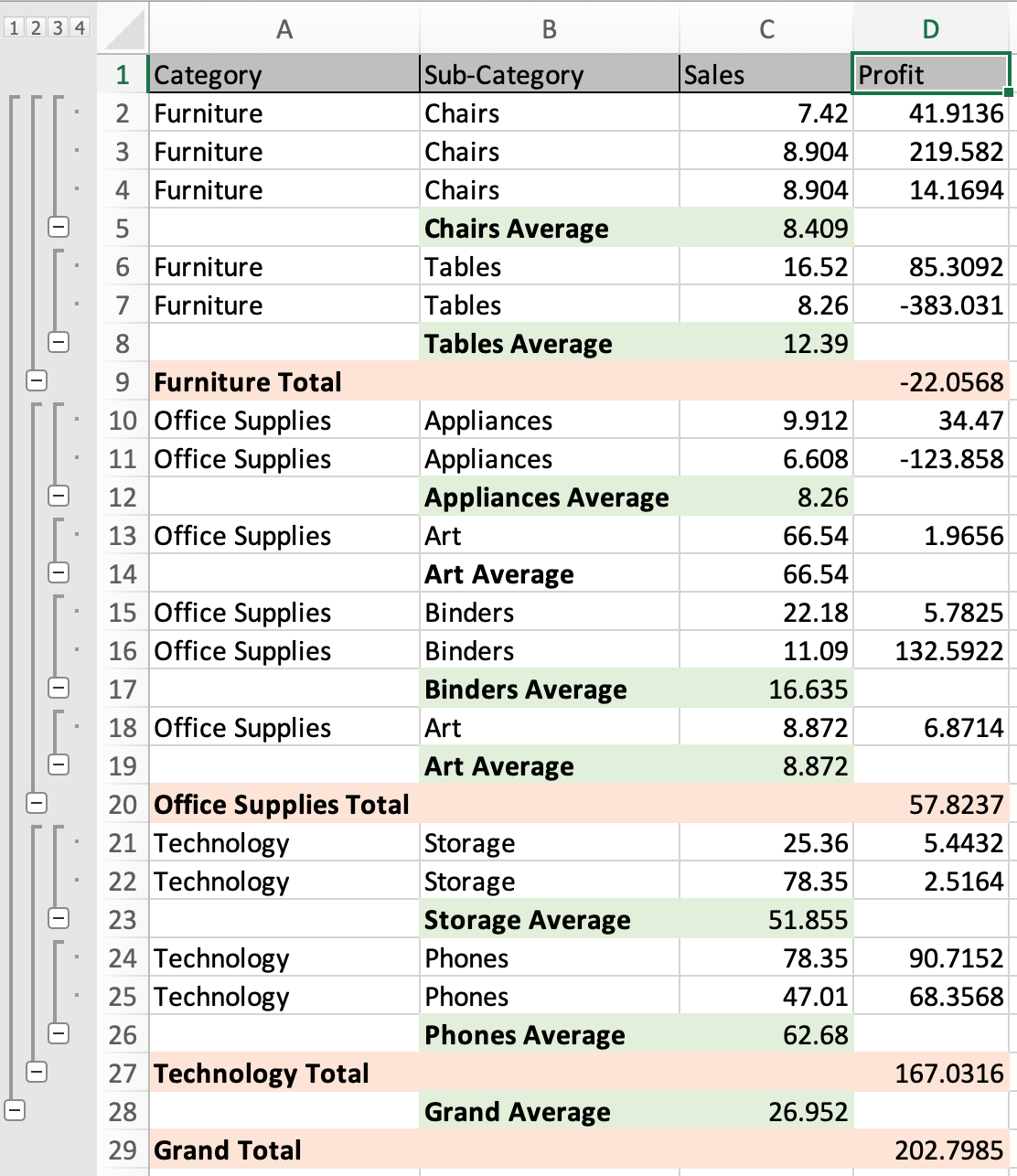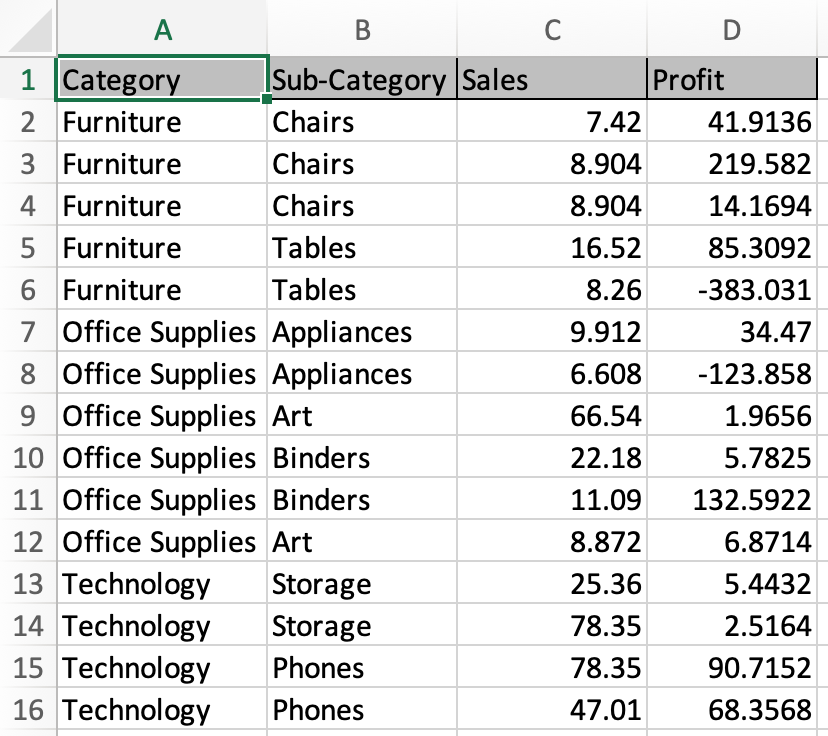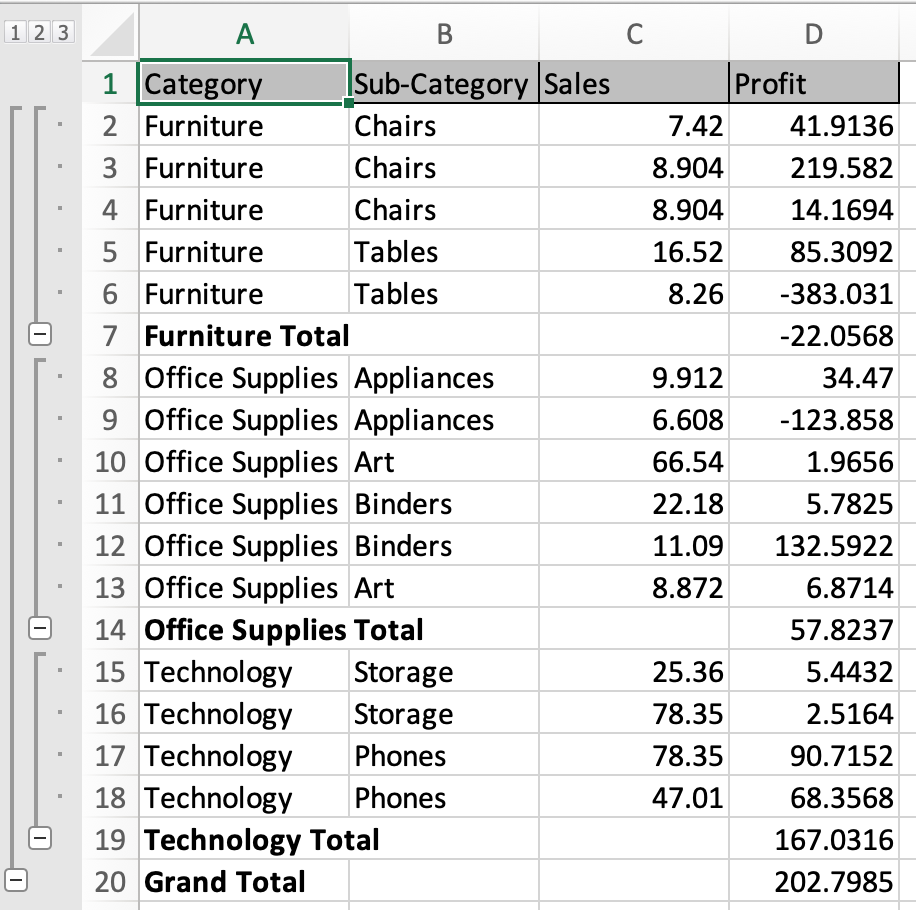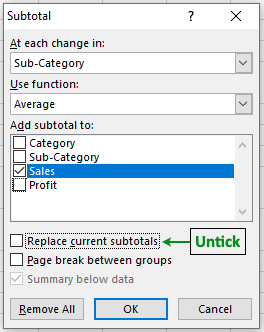Scenario:

Problem here is how we use more than one function in subtotaling data. For example Getting the average commute time of employees with the sum of employee daily expense basis on each department. For these

Multiple subtotal functions in Excel

Select the table > Go to Data > Subtotal > Select the first grouping column > first aggregate function > first subtotal column > Click Ok.

Select the table > Go to Data > Subtotal > Select the second grouping column > second aggregate function > second subtotal column > Untick Replace current subtotal box > Click Ok.

Continue till the data is grouped with different subtotals

Example :

All of these might be confusing to understand. Let's understand how to use the function using an example. Here we have product sales and profit data.We will first group data based on the month. Select the table and Go to Data > Subtotal.

Subtotal dialog box appears in front.Select the Category column name > Choose Sum function > Profit column to subtotal > Click OK.You can see the category wise subtotal data in Excel. To add more subtotal.

Select the table again and Go to Data> Subtotal > Select Sub-category column name > Choose Average function > Sales column to subtotal > Untick Replace current Subtotal > Click Ok.Your data is categorized into sub groups sum category group and average subcategory group.Above snapshot, green coloured are subcategory grouped subtotal and orange ones are Category grouped subtotal. You can also perform this using the pivot tables. Learn more about pivot tables here.

Here are all the observational notes using the formula in Excel
Notes :

1. Select the data before proceeding to the subtotal option. Or else the function throws an error.
2. To remove subtotal. Select table and Go to Data > subtotal > click Remove all button (adjacent to Ok button).

Hope this article about How to Add Additional Functions to Subtotals in Excel is explanatory. Find more articles on calculating values and related Excel formulas here. If you liked our blogs, share it with your friends on Facebook. And also you can follow us on Twitter and Facebook. We would love to hear from you, do let us know how we can improve, complement or innovate our work and make it better for you. Write to us at info@exceltip.com.

Related Articles :

How to Select Entire Column and Row Using Keyboard Shortcuts in Excel : Selecting cells is a very common function in Excel. Use Ctrl + Space to select columns and Shift + Space to select rows in Excel.

How do I Insert a Checkmark in Excel 2016 : To insert a checkmark in Excel Cell we use the symbols in Excel. Set the fonts to wingdings and use the formula Char(252) to get the symbol of a check mark.

How to disable Scroll Lock in Excel : Arrow keys in excel move your cell up, down, Left & Right. But this feature is only applicable when Scroll Lock in Excel is disabled.

Scroll Lock in Excel is used to scroll up, down, left & right your worksheet not the cell. So this article will help you how to check scroll lock status and how to disable it?
Excel Formula to Sort Text : To sort text values using formula in excel we simply use the COUNTIF function. Here is the formula

How to use the SUBTOTAL function in Excel: Returns the aggregate function SUM, COUNT, AVERAGE, STDEV or PRODUCT on applied filtered data in Excel.

Popular Articles :

How to use the IF Function in Excel : The IF statement in Excel checks the condition and returns a specific value if the condition is TRUE or returns another specific value if FALSE.

How to use the VLOOKUP Function in Excel : This is one of the most used and popular functions of excel that is used to lookup value from different ranges and sheets.

How to use the SUMIF Function in Excel : This is another dashboard essential function. This helps you sum up values on specific conditions.

How to use the COUNTIF Function in Excel : Count values with conditions using this amazing function. You don't need to filter your data to count specific values. Countif function is essential to prepare your dashboard.

Terms and Conditions of use

The applications/code on this site are distributed as is and without warranties or liability. In no event shall the owner of the copyrights, or the authors of the applications/code be liable for any loss of profit, any problems or any damage resulting from the use or evaluation of the applications/code.